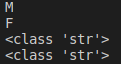# Strings in Python

## Strings in Python with Examples

In this article, I am going to discuss Strings in Python with Examples. Please read our previous article where we discussed Looping Statements in Python with examples. At the end of this article, you will understand the following pointers in detail which are related to python string.

1. What is a string in Python?
2. What is Slicing in Python?
3. What is Immutable in Python?
4. Strings are immutable in Python
5. Mathematical operators on string objects in Python
6. How to Find the Length of a string in Python?
7. How to remove spaces from a string in Python?
8. How to Find substrings in a string in python?
9. How to Count substring in a given String in Python?
10. How to replace a string with another string in Python?
11. Does replace() method modify the string objects in python?
12. How to Split a string in Python?
13. How to Join strings in Python?
14. How to change cases of string in Python?
15. How to Formatting the Strings in Python?
##### Reminder

We already learn the first hello world program in python. In that program, we just print a group of characters by using the print() function. Those groups of characters are called as a string.

Program to print string (Demo1.py)
print(“Welcome to python programming”)
Output: Welcome to python programming

##### What is a string in Python?

A group of characters enclosed within single or double or triple quotes is called a string. We can say the string is a sequential collection of characters.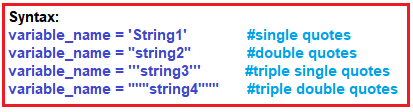##### Program to print employee information (Demo2.py)
```name = "Balayya"
emp_id = 20
print("Name of the employee: ", name)
print("employee id is :", emp_id)```
###### Output: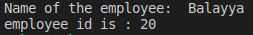Note: Generally, to create a string mostly used syntax is double quotes syntax.

##### When triple single and triple-double quotes are used?

If you want to create multiple lines of string, then triple single or triple-double quotes are the best to use.

###### Program to print multi-line employee information (Demo3.py)
```loc1 = """XYZ company
While Field
Bangalore"""
loc2 = """XYZ company
Banglore
Human tech park"""
print(loc1)
print(loc2)```
###### Output: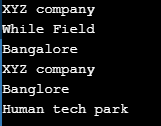Note: Inside string, we can use single and double quotes

##### Program to use single and double quotes inside a string (Demo4.py)
```s1 = "Welcome to 'python' learning"
s2 = 'Welcome to "python" learning'
s3 = """Welcome to 'python' learning"""
print(s1)
print(s2)
print(s3)```
###### Output: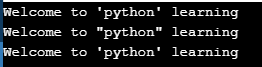##### The Empty string in Python:

If a string has no characters in it then it is called an empty string.

###### Program to print an empty string (Demo5.py)
```s1 = ""
print(s1)```

Output:

##### Accessing string characters in python:

We can access string characters in python by using,

1. Indexing
2. Slicing
##### Indexing:

Indexing means a position of string’s characters where it stores. We need to use square brackets [] to access the string index. String indexing result is string type. String indices should be integer otherwise we will get an error. We can access the index within the index range otherwise we will get an error.

##### Python supports two types of indexing
1. Positive indexing: The position of string characters can be a positive index from left to right direction (we can say forward direction). In this way, the starting position is 0 (zero).
2. Negative indexing: The position of string characters can be negative indexes from right to left direction (we can say backward direction). In this way, the starting position is -1 (minus one).
###### Diagram representation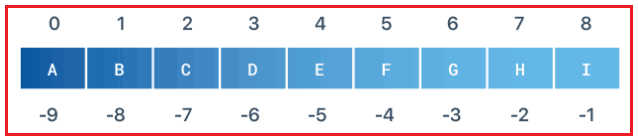Note: If we are trying to access characters of a string without of range index, then we will get an error as IndexError.

##### Example: Accessing a string with index in Python (Demo6.py)
```wish = "Hello World"
print(wish)
print(wish)```

Output: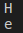###### Example: Accessing a string with float index in Python (Demo7.py)
```wish = "Hello World"
print(wish[1.3])```
###### Output: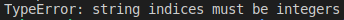##### Example: Accessing a string without of bound index (Demo8.py)
```wish = "Hello World"
print(wish)```
###### Output: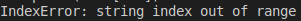###### Example: Accessing a string with a negative index (Demo9.py)
```wish = "Hello World"
print(wish[-1])
print(wish[-2])```
###### Output: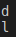##### Example: Printing character by character using for loop (Demo10.py)
```name ="Python"
for a in name:
print(a)```
###### Output: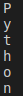##### What is Slicing in Python?

A substring of a string is called a slice. A slice can represent a part of a string from a string or a piece of string. The string slicing result is string type. We need to use square brackets [] in slicing. In slicing, we will not get any Index out-of-range exception. In slicing indices should be integer or None or __index__ method otherwise we will get errors.

###### Syntax: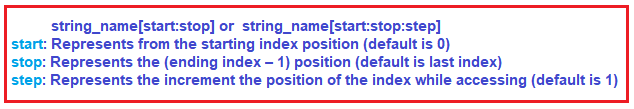###### Example: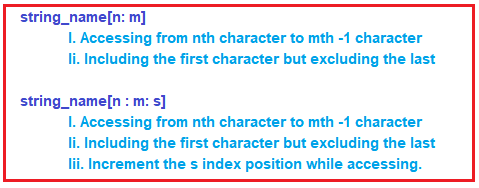##### Different Cases:

wish = “Hello World”

1. wish [::] => accessing from 0th to last
2. wish [:] => accessing from 0th to last
3. wish [0:9:1] => accessing string from 0th to 8th means (9-1) element.
4. wish [0:9:2] => accessing string from 0th to 8th means (9-1) element.
5. wish [2:4:1] => accessing from 2nd to 3rd characters.
6. wish [:: 2] => accessing entire in steps of 2
7. wish [2 ::] => accessing from str to ending
8. wish [:4:] => accessing from 0th to 3 in steps of 1
9. wish [-4: -1] => access -4 to -1

Note: If you are not specifying the beginning index, then it will consider the beginning of the string. If you are not specifying the end index, then it will consider the end of the string. The default value for step is 1

##### Example: Slicing operator using several use cases (Demo11.py)
```wish = "Hello World"
print(wish[::])
print(wish[:])
print(wish[0:9:1])
print(wish[0:9:2])
print(wish[2:4:1])
print(wish[::2])
print(wish[2::])
print(wish[:4:])
print(wish[-4:-1])```
###### Output: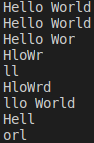##### What is Immutable in Python?

Once we create an object then the state of the existing object cannot be changed or modified. This behavior is called immutability. Once we create an object then the state of the existing object can be changed or modified. This behavior is called mutability.

##### Strings are immutable in Python:

A string having immutable nature. Once we create a string object then we cannot change or modify the existing object.

###### Example: Immutable (Demo12.py)
```name = "Balayya"
print(name)
print(name)
name="X"```

Output: TypeError: ‘str’ object does not support item assignment

##### Mathematical operators on string objects in Python

We can perform two mathematical operators on a string. Those operators are:

2. Multiplication (*) operator.
##### Addition operator on strings in Python:

The + operator works like concatenation or joins the strings. While using the + operator on the string then compulsory both arguments should be string type, otherwise, we will get an error.

###### Example: Concatenation (Demo13.py)
```a = "Python"
b = "Programming"
print(a+b)```

Output: Python Programming

##### Example: Concatenation (Demo14.py)
```a = "Python"
b = 4
print(a+b)```
###### Output: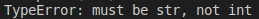##### Multiplication operator on Strings in Python:

This is used for string repetition. While using the * operator on the string then the compulsory one argument should be a string and other arguments should be int type.

###### Example: Multiplication (Demo15.py)
```a = "Python"
b = 3
print(a*b)```

Output: PythonPythonPython

##### Example: Multiplication (Demo16.py)
```a = "Python"
b = "3"
print(a*b)```
###### Output: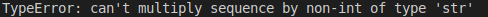##### How to Find the Length of a string in Python?

We can find the length of the string by using the len() function. By using the len() function we can find groups of characters present in a string. The len() function returns int as result.

###### Example: len Function (Demo17.py)
```course = "Python"
print("Length of string is:",len(course))```

Output: Length of string is: 6

##### Membership Operators in Python:

We can check, if a string or character is a member/substring of string or not by using the below operators:

1. In
2. not in
##### in operator

in operator returns True, if the string or character found in the main string.

###### Example: in operator (Demo18.py)
```print('p' in 'python')
print('z' in 'python')
print('on' in 'python')
print('pa' in 'python')```
###### Output: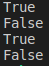##### Example: To find substring in the main string (Demo19.py)
```main=input("Enter main string:")
s=input("Enter substring:")
if s in main:
print(s, "is found in main string")
else:
###### Output1: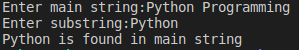###### Output2: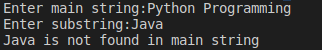##### Not in Operator in Python

The not-in operator returns the opposite result of in operator. not in operator returns True, if the string or character is not found in the main string.

Example: not in operator (Demo20.py)
print(‘b’ not in ‘apple’)
Output: True

##### Comparing strings in Python:

We can use the relational operators like >, >=, <, <=, ==, != to compare two string objects. While comparing it returns boolean values, either True or False. The comparison will be done based on alphabetical order or dictionary order.

###### Example: Comparison operators on the string in Python (Demo21.py)
```s1 = "abcd"
s2 = "abcdefg"
print(s1 == s2)
if(s1 == s2):
print("Both are same")
else:
print("not same")```
###### Output: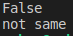###### Example: User name validation (Demo22.py)
```user_name ="rahul"
name = input("Please enter user name:")
if user_name == name:
print("Welcome to gmail: ", name)
else:
print("Invalid user name, please try again")```
###### Output: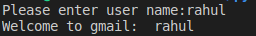##### How to remove spaces from a string in Python?

Space is also considered as a character inside a string. Sometimes unnecessary spaces in a string will lead to wrong results.

###### Example: To check space importance in the string at the end of the string (Demo23.py)
```s1 = "abcd"
s2 = "abcd    "
print(s1 == s2)
if(s1 == s2):
print("Both are same")
else:
print("not same")```
###### Output: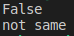##### Predefined methods to remove spaces in Python
1. rstrip() -> remove spaces at right side of string
2. lstrip() -> remove spaces at left side of string
3. strip() -> remove spaces at left & right sides of string

Note: These methods won’t remove the spaces which are in the middle of the string.

##### Example: To remove spaces in the star and end of the string in python (Demo24.py)
```course = "Python  "
print("with spaces course length is: ",len(course))
x=course.strip()
print("after removing spaces, course length is: ",len(x))```
###### Output: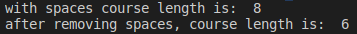##### How to Find substrings in a string in python?

We can find a substring in two directions like forwarding and backward direction. We can use the following 4 methods:

1. find()
2. index()
1. rfind()
2. rindex()
##### find() method:

Syntaxnameofthestring.find(substring)

This method returns an index of the first occurrence of the given substring, if it is not available, then we will get a -1(minus one) value. By default find() method can search the total string of the object. You can also specify the boundaries while searching.

Syntax – nameofthestring.find(substring, begin, end)

It will always search from beginning index to end-1 index.

##### Example: finding substring by using find method in python (Demo25.py)
```course="Python programming language"
print(course.find("p"))
print(course.find("a", 1, 20))```
###### Output: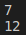##### index() method in Python:

index() method is exactly the same as the find() method except that if the specified substring is not available then we will get ValueError. So, we can handle this error at the application level

###### Example: finding substring by using index method (Demo26.py)
```s="Python programming language"
print(s.index("Python"))```

Output: 0

###### Example: finding substring by using index method (Demo27.py)
```s="Python programming language"
print(s.index("Hello"))```
###### Output: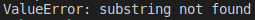###### Example: Handling the error (Demo28.py)
```s="Python programming language"
try:
print(s.index("Hello"))
except ValueError:

##### How to Count substring in a given String in Python?

By using the count() method we can find the number of occurrences of substring present in the string

Syntax – nameofthestring.count(substring)

###### Example: count() (Demo29.py)
```s="Python programming language, Python is easy"
print(s.count("Python"))
print(s.count("Hello"))```
###### Output: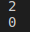##### How to replace a string with another string in Python?

We can replace the old string with a new string by using replace() method. As we know string is immutable, so replace() method never performs changes on the existing string object. The replace() method creates a new string object, we can check this by using the id() method.

Syntax- nameofthestring.replace(old string, new string)

###### Example: replace a string with another string (Demo30.py)
```s1="Java programming language"
s2=s1.replace("Java", "Python")
print(s1)
print(s2)
print(id(s1))
print(id(s2))```
###### Output: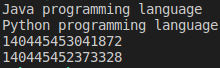##### Does replace() method modify the string objects in python?

A big NO. The string is immutable. Once we create a string object, we cannot change or modify the existing string object. This behavior is called immutability.

If you are trying to change or modify the existing string object, then with those changes a new string object will be created. So, replace the () method will create a new string object with the modifications.

##### How to Split a string in Python?

We can split the given string according to the specified separator by using the split() method. The default separator is space. split() method returns list.

Syntax- nameofthestring=s.split(separator)

###### Example: Splitting a string in Python (Demo31.py)
```message="Python programming language"
n=message.split()
print("Before splitting: ",message)
print("After splitting: ",n)
print(type(n))
for x in n:
print(x)```
###### Output: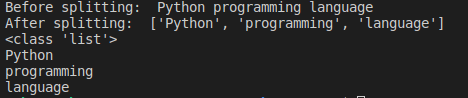###### Example: Splitting a string based on separator in python (Demo32.py)
```message="Python programming language,Python is easy"
n=message.split(",")
print(n)```
###### Output: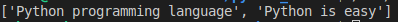##### How to Join strings in Python?

We can join a group of strings (list or tuple) with respect to the given separator.

Syntax: s=separator.join(group of strings)

###### Example: separator is – symbol joining string by using join() method (Demo33.py)
```profile = ['Roshan', 'Actor', 'India']
candidate = '-'.join(profile)
print(profile)
print(candidate)```
###### Output: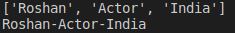###### Example: separator is : symbol joining string by using join() method (Demo34.py)
```profile = ['Roshan', 'Actor', 'India']
candidate = ':'.join(profile)
print(profile)
print(candidate)```
###### Output: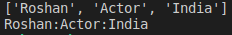##### How to change cases of string in Python?
1. upper() – This method converts all characters into upper case
2. lower() – This method converts all characters into lower case
3. swapcase() – This method converts all lower-case characters to uppercase and all upper-case characters to lowercase
4. title() – This method converts all character to title case (The first character in every word will be in upper case and all remaining characters will be in lower case)
5. capitalize() – Only the first character will be converted to upper case and all remaining characters can be converted to lowercase.
##### Example: Changing cases (Demo35.py)
```message='Python programming language'
print(message.upper())
print(message.lower())
print(message.swapcase())
print(message.title())
print(message.capitalize())```
###### Output: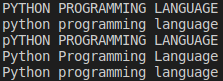##### How to Formatting the Strings in Python?

We can format the strings with variable values by using replacement operator {} and format() method.

###### Example: Formatting strings (Demo36.py)
```name='Rakesh'
salary=100
age=16
print("{} 's salary is {} and his age is {}".format(name, salary, age))
print("{0} 's salary is {1} and his age is {2}".format(name, salary, age))
print("{x} 's salary is {y} and his age is{z}".format(z=age,y=salary,x=name))```
###### Output: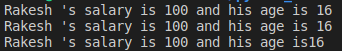##### Character Data Type in Python:

If a single character stands alone then we can say that is a single character. For example: M -> Male F -> Female. In other programming languages, char data type represents the character type, but in python, there is no specific data type to represent characters. We can fulfill this requirement by taking a single character in single quotes. A single character also represents a string type in python.

###### Example: Character data type (Demo37.py)
```gender1 = "M"
gender2 = "F"
print(gender1)
print(gender2)
print(type(gender1))
print(type(gender2))```
###### Output: